Create a new printableGeometry
Math Worksheets

Sample - Click above to make a new math worksheet (PDF).
 Name _____________________________Date ___________________
Coordinate Plane
Write the coordinates for each point.
1.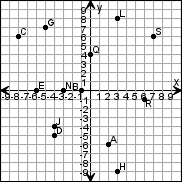G _______

 J _______

 A _______

 B _______

 C _______
2.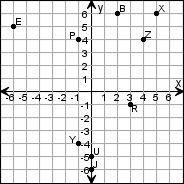J _______

 X _______

 Y _______

 U _______

 B _______

Find each ordered pair. Write the letter for the point named by the ordered pair.
3.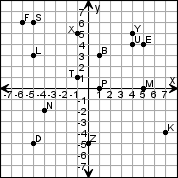(1, 3) _______

 (0, -5) _______

 (1, 0) _______

 (-6, 6) _______

 (5, 4) _______
4.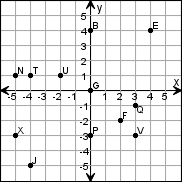(4, 4) _______

 (-2, 1) _______

 (0, -3) _______

 (2, -2) _______

 (-5, -3) _______

Draw the ordered points on the grid. Label each point.
5.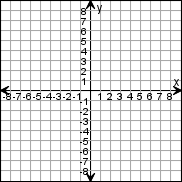L  (-2, -3)X  (1, 8)U  (-7, 3)K  (5, 4)D  (0, -8)T  (0, 0)
6.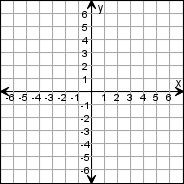B  (6, 3)P  (-2, -3)X  (-3, 0)U  (-3, -4)K  (4, -5)Z  (1, 0)

 Key #2
 aKey #2
Name the quadrant or on which axis the point lies.
7.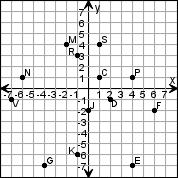S _______

 E _______

 M _______

 G _______

 F _______
8.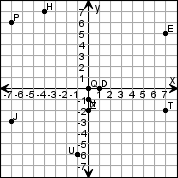U _______

 N _______

 J _______

 Q _______

 P _______

Complete the function table.
9.
y = x - 6
 x y 0 1 2 3
10.
y = 4x - 8
 x y 0 1 2 3
11.
y = 2x - 1
 x y -3 -2 -1 0

Complete the function table and then graph the function.
12.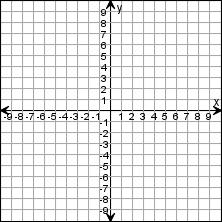y = x - 2
 x y -3 -2 -1 0
13.y = 7 + 3x
 x y -3 -2 -1 0

 Key #2
 aKey #2
Make a function table for the line. Write an equation for this function.
14.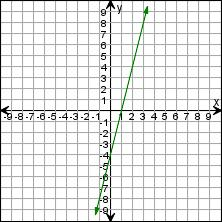x y
15.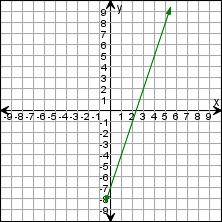x y

Draw the ordered points on the grid. Label each point.
16.G  (-3, 4)P  (2, -4)J  (0, 0)H  (-5, 3)M  (0, -6)T  (5, -3)
17.T  (-7, 8)A  (-1, 7)X  (-3, -2)E  (-5, -1)L  (1, -3)F  (7, 5)

Name the quadrant or on which axis the point lies.
18.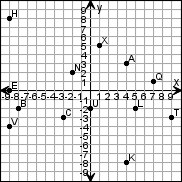T _______

 X _______

 L _______

 C _______

 U _______
19.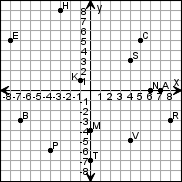P _______

 A _______

 C _______

 M _______

 N _______